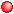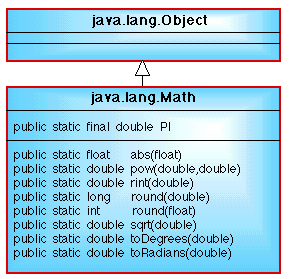# NOTE: This set of www pages is not the set of www pages for the curent version of COMP101. The pages are from a previous version that, at the request of students, I have kept on line.

## CONTENTS

 1. Overview of arithmetic operators 2. Example problem - circle calculation 2.1. Requirements 2.2. Analysis ` ` 2.3. Design 2.4. Implementation 2.5. Testing 3. Trigonometric ratios

In addition, in this lecture, we will be considering defining our own constructors and the use of constants (the PI constant from the Math class in this case). In the giant letters example we used the default constructor to create an instance of the class GiantLetters. In the circle calculation example given here (the Circle class) we will be defining our own constructor. The Circle class example will also illustrate: (1) the use of the Double wrapper class, and (2) the use of a programmer defined toString method (introduced when discussing the Integer wrapper class). Note also that the Circle class will form the "super class" when we consider inheritance in a later lecture.

## 1. OVERVIEW OF ARITHMETIC OPERATORS

Arithmetic operations are among the most fundamental instructions that can be included in a computer program. Java supports all the standard mathematics operations (see Table 1).

Note that:Multiplication is indicated by an asterisk *.Division is indicated by a forward slash /.The % operator will compute the remainder after the division of two integer values. For example 11%2 will give 1 while 2%11 will give 2.
OperatorInterpretation
-Unary minus or subtraction
*Multiplication
/Division
%Remainder
++Incrementation (by 1)
--Decrementation (by 1)
-=Subtract expression

Table 1: Arithmetic operatorsWhen dividing one integer number (short, int or long) by another the result is always an integer. For example 5/2 will be 2 and not 2.5!The ++ and --, incrementation and decrementation operators, are equivalent to add or subtract one to a numeric data item. For example number++ and number-- (or ++number and --number) are equivalent to: ```number = number+1; number = number-1; ``` respectively.The += and -= are used to add/subtract an arithmetic expression to the value of a data item. For example: ```number = number+(6/2); number = number-(6/2); ``` will be equivalent to: ```number += 6/2; number -= 6/2; ```The ++, --, += and -= are provided as "short cuts" for frequently required arithmetic operations.

More complex arithmetic operations can be found in the Java Math class. A fragment of the Math class is given in Figure 1. The class is contained in the java.lang package which is always included in all Java programs. The class includes constants such as PI and methods such as:

` `abs(float) returns the absolute value of its argument (for example Math.abs(-12.3) will return 12.3. There are abs methods for each of the primitive numeric types double, float, int and long.pow(double,double) which returns the value of its first argument raised to the power of the second argument. Note that both arguments are doubles.Figure 1: Math class diagram showing the fields and methods referenced heresqrt(double) which returns the square root of its argument (a double).round(double) and round(float) which return the closest long integer to the argument and the closest standard integer to the argument respectively.rint(double) returns the closest "integer" to its argument, but as a data item of type double.

These methods are all class (static) methods and thus we do not need to create an instance of the class Maths to use them --- we simply link them to the class name. For example:

```Math.abs(number);
```

where number is a data item of type integer.

### 1.1. Note on e-notation

Values for variables are sometimes expressed using what is known as or scientific notation/format. For example:

```0.2e004
```

or:

```0.2e-06
```

where the e (standing for exponent) separates the number from the exponent. Thus 0.2e004 is interpreted as 0.2x10^4 which equals 0.2x10000 which equals 2000. The above example value of 0.2e-06 is then equivalent to 0.2x10^-6 which equals 0.2x0.000001 which equals 0.0000001.

## 2. EXAMPLE PROBLEM --- CIRCLE CALCULATION

### 2.2. Analysis

Using "noun extraction" the class diagram presented in Figure 3 is proposed.

### 2.3. Design

From Figure 3 the design comprises two classes: Circle and CircleApp.

#### 2.3.1. Circle ClassFigure 3: Circle class diagram

 Field Summary private double circleRadius            An instance field to store the radius of an instance of the class Circle. private double circleCircum            An instance field to store the circumference of an instance of the class Circle. private double circleArea            An instance field to store the area of an instance of the class Circle.

 Constructor Summary Circle(int newRadius)            Constructs an instance of the class Circle given a radius as a formal parameter (newRadius) which is assigned to the instance varaiable circleRadius.

 Method Summary public void calcCircleCircum()            Instance method to calculate the circumference of a circle using the circleRadius instance variable and assign the result to the instance variable circleCircum. Method makes use of the constant PI held in the Math class. public void calcCircleArea()            Instance method to calculate the area of a circle using the circleRadius instance variable and assign the result to the instance variable circleArea. Method makes use of the constant PI held in the Math class. public String toString()            Instance method to return output string for circle circumference and area instance fields.

Nassi-Shneiderman charts for all of the above methods are presented in Figure 4.Figure 4: Nassi-Shneiderman charts for Circle class methods

#### 2.3.2. CircleApp Class

 Field Summary private static Scanner input            A class instance to facilitate input from the keyboard.

 Method Summary public static void main(String[] args)            Main method to allow input of circle radius, calls to calcCircleCircum, calculateCircleAera and toString public Circle instance methods.

A Nassi-Shneiderman for the above is presented in Figure 5.Figure 5: Nassi-Shneiderman charts for CircleApp class method

### 2.4. Implementation

#### 2.4.1. Circle class

 ```// CIRCLE CLASS // Frans Coenen // Tuesday 2 March 1999 // The University of Liverpool, UK class Circle { // ------------------- FIELDS ------------------------ private double circleRadius; private double circleCircum; private double circleArea; // ------------------ CONSTRUCTORS ------------------- /* Constructor */ public Circle(double newRadius) { circleRadius = newRadius; } // ------------------ METHODS ------------------------ /* Calculate circumference */ public void calcCircleCircum() { circleCircum = 2.0*Math.PI*circleRadius; } /* Calculate area */ public void calcCircleArea() { circleArea = Math.PI*circleRadius*circleRadius; } /* Output circumference and area */ public String toString() { return("Circumference = " + circleCircum + "\nArea = " + circleArea + "\n"); } } ```

Table 2: Circle Class definition

#### 2.4.2. CircleApp class

 ```// CIRCLE APPLICATION CLASS // Frans Coenen // Tuesday 2 March 1999 // Revised: Friday 29 March 2005, Thursday 12 May 2005 // The University of Liverpool, UK import java.util.*; class CircleApp { // ------------------- FIELDS ------------------------ // Create instance of Scanner class private `static Scanner input = new Scanner(System.in); // ------------------ METHODS ------------------------ public static void main(String[] args) { // Input radius System.out.print("Input a radius (double): "); double radius = input.nextDouble(); // Create new Circle instance Circle newCircle = new Circle(radius); // Calculate circumference and area newCircle.calcCircleCircum(); newCircle.calcCircleArea(); // Output result System.out.println(newCircle); } } ```

Table 3: Circle Class application program

Note how the toString method is invoked in Table 3, we simply include the instance name as the argument in the println (or print) statement which causes the toString method to be invoked automatically.

Remember also that fields, regardless of whether they are private, protected or public, or whether they are class fields or instance fields, can always be used anywhere inside the class in which they are declared. However, data items declared as prt of a method definition, including data items declared as formal parameters (arguments) can only be used within the method in which they are declared.

### 2.5. Testing

Software testing encompasses a significant amount of the overall development effort.

Arithmetic Testing: The above is a fairly straightforward procedure and thus very little testing is required. Given an arithmetic statement it is always a good idea to test the effect that negative, positive and zero sample values will have on the outcome. In this case there is only one numeric input, we should therefore test negative and positive inputs as well as zero. If there is more than one input we should test every possible combination of positive, negative and zero input. Thus if we have two inputs we would have 3x3 possible combinations, with three inputs 3x3x3 possible combinations (and so on). This form of testing is known as arithmetic testing.

We do this testing by generating a set of test cases, 3 in this case, as illustrated in the table to the right. Note that when we input a negative number we produce a negative circumference - this is probably not the desired result and thus we should return to the requirements phase and determine what action we should take in the event of a negative radius --- (a) error, (b) convert to a positive number, (c) ... ? --- however our programming skills are at present such that we just have to "live with this undesirable result".

TEST CASEEXPECTED RESULT
10.062.831853314.159
0.00.00000.0000
-10.0-62.831853314.159

Data Validation: A second important testing method is known as Data validation testing. Here we provide input data with incorrect syntax, both blatant and subtle. A suggested set of test cases are presented below. The aim of these tests is to insure that the program exits cleanly with causing the system to crash.

TEST CASEEXPECTED RESULT
` `
 Methods that return the trigonometric ratios (sine, cosine, tangent, cosecant, secant and cotangent) given an appropriate angle are all also available in the Math class. However, it should be noted that the required angle for these methods must be presented in radians and not degrees. Given an angle D (degrees) we can convert this to an angle R (radians) as follows: ```R = D * Math.PI/180.0 ``` i.e. multiply by Math.PI/180.0. To convert from radians to degree we multiply by 180.0/Math.PI. Thus: ```D = R * 180.0/Math.PI ``` ` ` As might be expected, this is such a common operation that the Math class contains methods to do this: static double toDegrees(double angrad), converts an angle measured in radians to the equivalent angle measured in degrees. static double toRadians(double angdeg), converts an angle measured in degrees to the equivalent angle measured in radians. Note that in both cases the argument and the return value are doubles. Note also that these are class methods. An additional example problem that makes use of the trigonometric ratio methods described here is available.